Blog
Deep Learning
Machine Learning
Python
R&D

# Handwritten text recognition: A robust python pre-rendering pipeline for online handwritten text datasets (IAM) with numpy augmentations

7
min
Published on:
Aug 23, 2022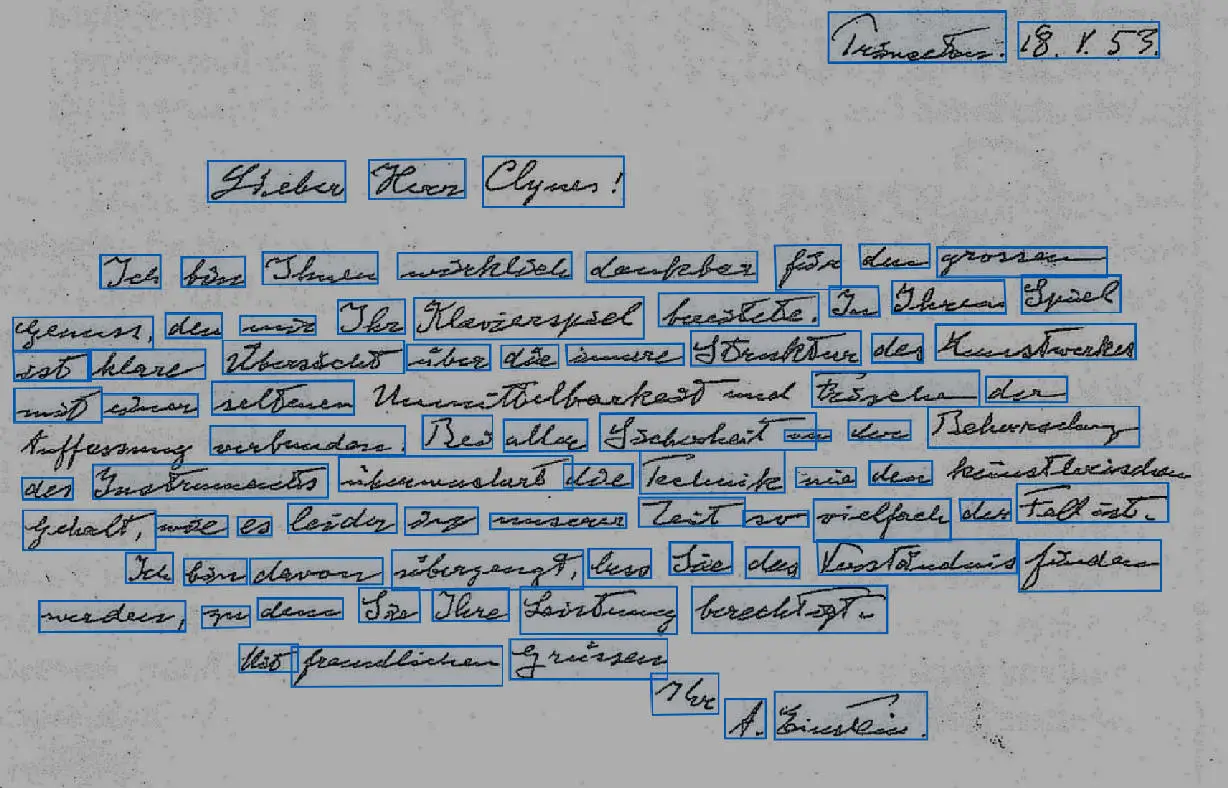Charles GaillardMohamed Biaz

Summary

Share the article

Offline handwritten text recognition datasets (optically scanned images), as opposed to online handwritten recognition datasets (record of the trajectory of the pen as a function of time), don’t contain images but strokes. We’ll try to explain how to create a pre-rendering pipeline for online handwritten that can be used for text recognition model training in python.

A stroke is a list of triplets (x, y, t) where (x, y) are the 2D coordinates of the points and (t) is the drawing time collected by the sensitive display, like a device with a touchscreen.

When training a text recognition model, we usually consider using datasets containing images because we use vision-based models. That’s why, most of the time, deep learning engineers orient themselves towards offline datasets and simply train their models with images and labels straight from the dataset, with image augmentations.

We must overcome this dependency on images: training a vision-based model by taking images as input does not necessarily require an image dataset. Online datasets contain a huge amount of precious data, which can be easily exploited and converted to images instantly. In addition, having access to the raw points of each stroke of each word, when using online datasets, allows us to perform a lot of NumPy operations directly on those points.

In this article, we will provide an entire python transformation pipeline for online handwritten datasets using IAM, starting with data points (strokes) to image rendering. It will include a collection of simple and fast Numpy augmentations performed directly on strokes and points.

## Image rendering for online handwritten recognition datasets using IAM

Before we get started, it is important to note that these operations are carried out on points and not images, which makes it extremely fast and only requires Numpy dependency.

IAM online text data is given as an XML file. We need to parse it to get the strokes. Below is a Python code snippet on how to parse an XML data point of the IAM offline dataset:

``````import xml.etree.ElementTree as ET
import numpy as np
import random

def parse_strokes(xml_path: str) -> List[np.ndarray]:
"""Parse a XML file from the IAM online dataset, returns a list of strokes (each one is an array of 2D points)"""
tree = ET.parse(xml_path)
root = tree.getroot()
strokes = [
[
(
int(point.attrib["x"]),
int(point.attrib["y"])
)
for point in stroke
]
for stroke in root[-1]
]
return [np.asarray(stroke) for stroke in strokes]
``````

If we simply draw the points on a white canvas, we obtain the raw rendering shown in the image below. For clarity of the code, the next code examples contain only the points manipulations: the canvas drawing will be shown later in the article. Note that for the following examples, we will use the first datapoint from the IAM online dataset (lineStrokes-all/lineStrokes/a01/a01-000/a01-000u-01.xml).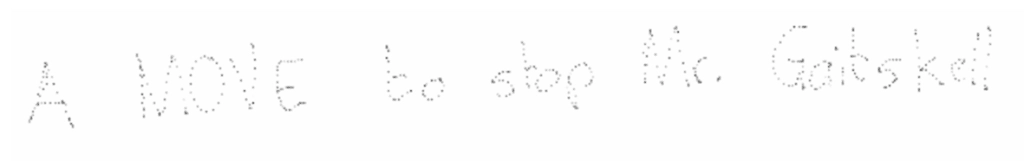Raw points of the first datapoint of IAM online plotted on a white canvas

It is that easy, but let’s not stop there. We can augment the resolution of points randomly, to avoid the “dashlane effect” (points instead of lines) and better distinguish letters:

``````def random_enrich_strokes(
strokes: List[np.ndarray],
max_factor: int = 3
) -> List[np.ndarray]:
"""Multiply by until 2 * max_factor the number of points in the strokes to have a better resolution."""
for _ in range(random.randint(1, max_factor)):
strokes = [
np.concatenate(
(p, [(p[i] + p[i + 1]) / 2 for i in range(len(p) - 1)]),
axis=0
)
for p in strokes
]
return strokes``````

This is how it renders if we multiply the number of points by a factor of 2: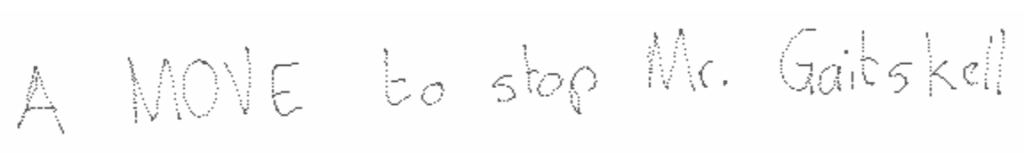Raw points of the first datapoint of IAM online plotted on a white canvas with two dots enrichment

The more we add points to the canvas, the more it looks like a plain line. This is important in case you want to train a handwritten text recognition model as it fits better a real data distribution. Here is an illustration to compare the two canvases without enrichment and with a factor of 2:

## Adding augmentation simulating real handwritten text

Let’s now perform random dilation (spacing them) on each stroke to displace letters relatively.

``````def random_dilate_strokes(
strokes: List[np.ndarray], x_d: float = 1e-3, y_d: float = 7e-2
) -> List[np.ndarray]:
"""Perform random vertical dilation on each stroke."""
# Compute random dilation parameters
y_dil = [random.uniform(1 - y_d, 1 + y_d) for _ in strokes]
x_dil = [random.uniform(1 - x_d, 1 + x_d) for _ in strokes]
return [
[(int(x_dil[i] * x), int(y_dil[i] * y)) for (x, y) in stroke]
for i, stroke in enumerate(strokes)
]``````

This is how it renders:

To operate on all points, let’s flatten the strokes in an array of points:

``````def flatten_strokes(strokes: List[np.ndarray]) -> np.ndarray:
"""Flatten a list of strokes in an array of points"""
return np.asarray([p for stroke in strokes for p in stroke], np.int32)
``````

Let’s now apply a random transformation to our points. The goal is to create variability in our data without adding new samples. We’ll add two transformations, shear, and rotation:

``````def random_transform_points(
points: np.ndarray, rot: float = 0.1, shear: float = 0.5
) -> np.ndarray:
"""Randomly transform 2D points"""
transform = np.asarray(
[
[random.uniform(1 - shear, 1 + shear), random.uniform(-rot, rot)],
[random.uniform(-rot, rot), random.uniform(1 - shear, 1 + shear)],
],
np.float32,
)
return np.matmul(points, transform)``````

We have 2 examples of the rendering here: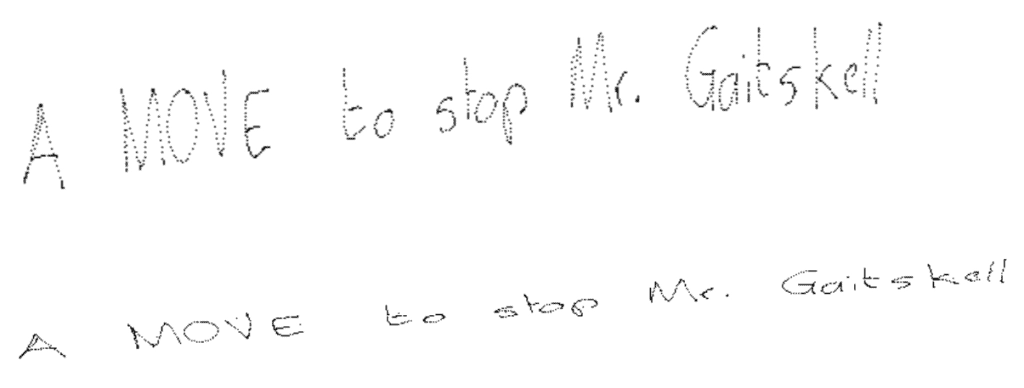Points are multiplied by a 2D transformation matrix before being plotted on the white canvas

Let’s resize points:

``````def resize_points(
points: np.ndarray, dwn_size: int = 10, shift: int = 10
) -> np.ndarray:
"""Downsize to have a decent image size, and shift to see full characters"""
points = points / dwn_size
points[:, 0] -= np.min(points[:, 0])
points[:, 1] -= np.min(points[:, 1])
return points + shift``````

Now all those manipulations may not be useful if we don’t render them, so we are now going to compute a Numpy canvas to draw the points on:

``````def compute_random_canvas(points: np.ndarray, shift: int = 10, noise: float = .5, light: float = .3) -> np.ndarray:
"""Compute the RGB canvas to fit the points."""
h, w = 2 * shift + int(np.max(points[:, 1])), 2 * shift + int(np.max(points[:, 0]))
# Compute canvas mode: uniform color or rainbow
canvas = (np.tile(np.arange(w), (h, 1)) / w) if random.random() > 0.5 else np.ones((h, w))
# Random reverse and roll each RGB channel
canvas = np.stack(
(
np.roll(canvas[..., ::-1], random.randint(0, w), 1) if random.random() > 0.5 else canvas,
np.roll(canvas[..., ::-1], random.randint(0, w), 1) if random.random() > 0.5 else canvas,
np.roll(canvas[..., ::-1], random.randint(0, w), 1) if random.random() > 0.5 else canvas,
),
axis=-1,
)
# Lighten
light = light * np.ones(canvas.shape)
# Compute noise
noise = random.uniform(0, noise) * np.random.rand(*canvas.shape)
return light + canvas - noise``````

These are generated samples of canvas:

Finally, let’s render our points on the canvas:

``````def random_draw(canvas: np.ndarray, points: np.ndarray, shift: int = 3, density: int = 20) -> np.ndarray:
"""Draw points with random local shifts and random colors on canvas.
"""
uniform_color = (random.random(), random.random(), random.random()) if random.random() > .5 else None
uniform_shift = np.random.randint(1, shift) if random.random() > .5 else None
for point in points:
x, y = point
color = uniform_color if uniform_color else (random.random(), random.random(), random.random())
if uniform_shift:
for i in range(uniform_shift):
for j in range(uniform_shift):
canvas[int(y) + i, int(x) + j] = color
else:
for _ in range(random.randint(1, density)):
canvas[int(y) + random.randint(0, shift), int(x) + random.randint(0, shift)] = color
return canvas``````

Here are some samples with drawing variations: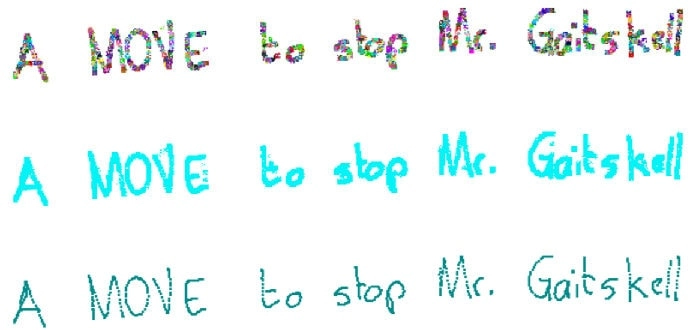Same datapoint plotted with random noise and shift around points and different colors

From here, it is easier to create a generative augmentation pipeline, taking  a file path as input and rendering random augmented versions of the original datapoint from the IAM online dataset:

``````def random_augment(filepath: str, n_samples: int = 100):
for _ in range(n_samples):
strokes = parse_strokes(filepath)
# Operations on strokes
strokes = random_enrich_strokes(strokes)
strokes = random_dilate_strokes(strokes)
points = flatten_strokes(strokes)
# Operations on points
points = random_transform_points(points)
points = resize_points(points)
# Draw on canvas
canvas = compute_random_canvas(points)
canvas = random_draw(canvas, points)``````

The next image contains 10 randomly generated samples done with the previous code snippet:

One can play with the parameters of each function in the pipeline to modify the transformations.

## Conclusion

Online handwritten datasets can be exploited to generate a lot of very different image samples with simple augmentations. Since you manipulate points instead of images it is way faster than using offline datasets, and we are not even mentioning the dataset size to download. In the end, this is quick and easy, and it will surely help your handwritten text recognition model converge if you use this augmented dataset.

Feel free to join our slack community if you want to go further!

Computer Vision
Deep Learning
Python## Schedule a meeting with one of our experts

Please provide the following information so we can connect you to the right teammate.

Oops! Something went wrong while submitting the form.